## Recommended Posts

Hello, is it even possible that Einsteins definition of simultaneity is incorrect? Based on his paper I wrote down a number of reasons why I think the definition is plain wrong. (The paper I quoted from you can find clicking the link below).

I. KINEMATICAL PART § 1. Definition of Simultaneity (p.3)

-"We arrive at a much more practical determination along the following line of thought.

If at the point A of space there is a clock, an observer at A can determine the time values of events in the immediate proximity of A by finding the positions of the hands which are simultaneous with these events. If there is at the point B of space another clock in all respects resembling the one at A, it is possible for an observer at B to determine the time values of events in the immediate neigh- bourhood of B. But it is not possible without further assumption to compare, in respect of time, an event at A with an event at B. We have so far defined only an “A time” and a “B time.” We have not defined a common “time” for A and B, for the latter cannot be defined at all unless we establish by definition that the “time” required by light to travel from A to B equals the “time” it requires to travel from B to A. Let a ray of light start at the “A time” tA from A towards B, let it at the “B time” tB be reflected at B in the direction of A, and arrive again at A at the “A time tA.”

In accordance with definition the two clocks synchronize if  t B − t A = t A − t B .

We assume that this definition of synchronism is free from contradictions, and possible for any number of points; and that the following relations are universally valid:

1. If the clock at B synchronizes with the clock at A, the clock at A synchronizes with the clock at B.

2. If the clock at A synchronizes with the clock at B and also with the clock at C, the clocks at B and C also synchronize with each other.

Thus with the help of certain imaginary physical experiments we have settled what is to be understood by synchronous stationary clocks located at different places, and have evidently obtained a definition of “simultaneous,” or “synchronous,” and of “time.” The “time” of an event is that which is given simultaneously with the event by a stationary clock located at the place of the event, this clock being synchronous, and indeed synchronous for all time determinations, with a specified stationary clock.”

Einsteins assumption that his definition is possible for any number of points and that the relations are universally valid, is untenable and so is his conclusion, because:

- If the clock at A synchronizes with the clock at B and also with a third clock at C, these clocks only synchronize under the condition that the distances in between all three clocks are equal. Or: It requires the clocks to be placed on the vertexes of an equilateral triangle.

- The mutual distances of more than three clocks can not be all equal when the clocks are placed on the same plane.

- If the clock placed at A synchronizes with the clock at B and also with the clock at C, a fourth clock placed at D only synchronizes with clock A, B and C when clock A, B, C and D are placed at the vertexes of a tetrahedron.

- (The setup requires the symmetry of A and B to both act as a light source and as a reflector from the start.)*

This brings us to:

§2 On Relativity of Lengths and Times.(page 4, second last alinea)

-“We imagine further that at the two ends A and B of the rod, clocks are placed which synchronize with the clocks of the stationary system, that is to say that their indications correspond at any instant to the “time of the stationary system” at the places where they happen to be. These clocks are therefore “synchronous in the stationary system.” -“

To synchronize the clocks at two ends A and B of the rod in motion, it requires a second rod in the stationary system. At the two ends of the stationary rod clock C and D are placed. This creates a situation of two parallel rods of equal length and a total of four clocks necessary to make a balanced symmetrical comparison between a stationary rod S and a rod M in motion.*

We imagine further that with each clock there is a moving observer, and that these observers apply to both clocks the criterion established in § 1 for the synchronization of two clocks.

The criterion established in §1 is:  t B − t A = t A − t B .” (page3)

“In agreement with experience we further assume the quantity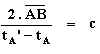to be a universal constant—the velocity of light in empty space.
It is essential to have time defined by means of stationary clocks in the stationary system, and the time now defined being appropriate to the stationary system we call it “the time of the stationary system.”

However if we apply the criterion established in §1 to 4 clocks on both ends of two stationary parallel rods, the following is valid:

t B − t A = t A − t B  =  t D− t B  = t B − t D = t C − t A = t A − t C

and

t B − t A = t A − t B     t D − t A = t A − t D = t C− t B  = t B − t C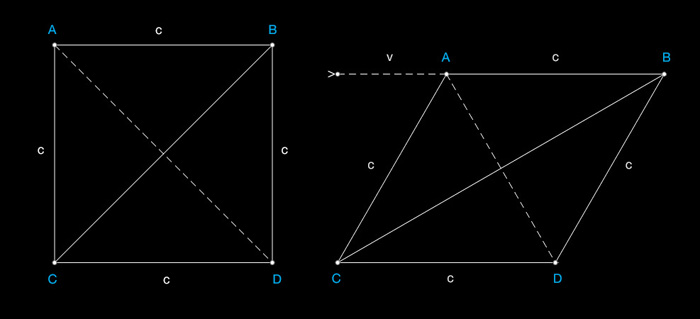This means that even when both rods are stationary compared to each other, it is still impossible to synchronize all four clocks using Einsteins criterion. Thus the following statement is also untenable.

Taking into consideration the principle of the constancy of the velocity of light we find that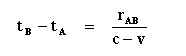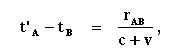where rAB denotes the length of the moving rod measured in the stationary system. Observers moving with the moving rod would thus find that the two clocks were not synchronous, while observers in the stationary system would declare the clocks to be synchronous.”,

The observers in the stationary system do not declare the clocks to be synchronous.

“So we see that we cannot attach any absolute signification to the concept of simultaneity, but that two events which, viewed from a system of co-ordinates, are simultaneous, can no longer be looked upon as simultaneous events when envisaged from a system which is in motion relatively to that system.

Even stationary events can only be looked upon as simultaneous events under the strict conditions I mentioned above. Temporarily conclusion: All the calculations in the next chapters of the KINEMATICAL PART concerningare based on a theoretically untenable thought experiment.

Thanks to Strange for the link ‎hermes.ffn.ub.es-luisnavarro-nuevo_maletin-Einstein_1905_relativity.pdf.webloc

Alternative Simultaneity: Placed at a vertex of a stationary equilateral triangle, for the observer of clocks at the opposite vertexes these clocks run synchronized. Observers placed at the opposite vertexes of an equilateral quadrangle having sides c will experience shared simultaneity via two clocks placed at the open vertexes. Under the condition that AD < 2c or BC < 2c simultaneity is preserved for both observers independently of their relative velocity. The diagram allows a straight forward derivation of the Lorentz Transformation and does not need time dilation. Important is that this setup is expandable and independent from inertial motion.

Edited by vanholten

##### Share on other sites
5 minutes ago, vanholten said:

If the clock at A synchronizes with the clock at B and also with a third clock at C, these clocks only synchronize under the condition that the distances in between all three clocks are equal.

Why?

I can't see how you reach that conclusion. Where does it say that the distances have to be equal?

##### Share on other sites
54 minutes ago, vanholten said:

If the clock at A synchronizes with the clock at B and also with a third clock at C, these clocks only synchronize under the condition that the distances in between all three clocks are equal. Or: It requires the clocks to be placed on the vertexes of an equilateral triangle.

No.  You have three clocks, A, B and C all at rest with respect to each other.  For this example we will put them in a straight line, with B between A and and twice as far from C as it is A.   When Clock B reads 12:00 it sends a light flash towards both A and C and then waits for the flash to reflect back to, and when each reflection returns, it records the reading it sees on each of the other clocks when its flash returns.

So if the flash from clock A returns when clock B reads 2:00,  B knows that clock A is 1 light hr away. And if the accompanying image of Clock A shows 1:00, Clock B knows that that image left 1 hr ago, clock A has advanced 1 hr in that time and at at the moment that B sees the returning flash reads 2:00. Thus clock A is in sync with clock B.

Further, the flash from clock C returns when clock B reads 4:00, meaning clock C is 2 light hrs away. If the accompanying image of Clock C reads 2:00, Clock B knows that it left 2 hrs ago, Clock C has advanced 2 hrs since then, reads 4:00 at the moment B sees the flash and is in sync with clock B. It also knows that Clock A has also advanced to read 4:00, so all three clocks are in sync.

While it is convenient to use equally spaced clocks to determine simultaneity, as it removes the need to determine the distance between clocks, it is not a requirement for determining simultaneity.

##### Share on other sites

2 hours ago, Strange said:

Why?

I can't see how you reach that conclusion. Where does it say that the distances have to be equal?

According to Einstein: "We have so far defined only an “A time” and a “B time.” We have not defined a common “time” for A and B, for the latter cannot be defined at all unless we establish by definition that the “time” required by light to travel from A to B equals the “time” it requires to travel from B to A. Let a ray of light start at the “A time” tA from A towards B, let it at the “B time” tB be reflected at B in the direction of A, and arrive again at A at the “A time tA.”

In accordance with definition the two clocks synchronize if  t B − t A = t A − t B ."

The definition of simultaneity implies that if C takes part in the simultaneity of A end B  the following should be true for clocks A, B and C:

t B − t A = t A − t B

and

t C− t A  = t A − t C

and

t B− t C = t C − t B

"§ 2. On the Relativity of Lengths and Times" (page 4:)  "velocity = light path/time interval"

Simultaneity between the stationary clocks depends on the velocity of light and requires equal time intervals. Equal time intervals require equal distances.

Edited by vanholten

##### Share on other sites
1 minute ago, vanholten said:

The definition of simultaneity implies that if C takes part in the simultaneity of A end B  the following should be true for clocks A, B and C:

t B − t A = t A − t B

and

t C− t A  = t A − t C

and

t B− t C = t B − t C

That is true. But it doesn't mean that tB - tA = tA - tC for example.

Janus has given a more detailed explanation, but it seems you don't understand the point Einstein is making. He is simply saying that the speed of light is the same in both directions. He isn't saying that all times have to be equal.

##### Share on other sites
47 minutes ago, Strange said:

That is true. But it doesn't mean that tB - tA = tA - tC for example.

Janus has given a more detailed explanation, but it seems you don't understand the point Einstein is making. He is simply saying that the speed of light is the same in both directions. He isn't saying that all times have to be equal.

Actually it does. If Janus would be right what is the point of simultaneity? Einstein is saying a lot more than that the speed of light is the same in both directions.  This is what Einstein wrote on page 2. the third alinea of Definition of Simultaneity:

"We have to take into account that all our judgments in which time plays a part are always judgments of simultaneous events."

This is true because simultaneity is an essential expression of the order of nature. Take the second law of Kepler for instance:

-A line segment joining a planet and the Sun sweeps out equal areas during equal intervals of time.

Isn't it amazingly wonderful?

You can't change the simultaneity of natural events by synchronizing manmade clocks to your own liking,  you have to look further than that.

Edited by vanholten

##### Share on other sites

Here is an extract on the subject from Einstein's own book on the subject.

He is much more chatty and less formal in the book, have you read it?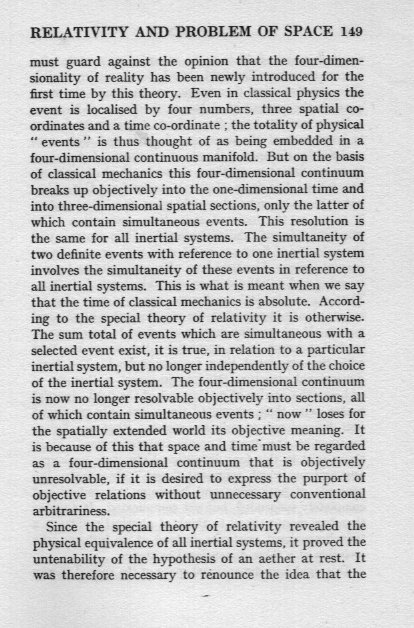##### Share on other sites
5 minutes ago, vanholten said:

Actually it does.

Why?

6 minutes ago, vanholten said:

You can't change the simultaneity of natural events by synchronizing manmade clocks to your own liking

No one is saying that. But there is no unique "simultaneity of natural events" that all observers will agree on.

##### Share on other sites
17 minutes ago, studiot said:

Here is an extract on the subject from Einstein's own book on the subject.

He is much more chatty and less formal in the book, have you read it?

No, I didn't. I only got really interested in the subject about a year ago. Thanks I will look into it.

Edited by vanholten

##### Share on other sites
Just now, vanholten said:

No, I didn't. I only got really got interested in the subject about a year.

You seem to have picked up an impressive number of misunderstandings in that time

##### Share on other sites

A short extract from the Preface

Quote

The present book is intended, as far as possible, to give an exact insight into the Theory of Relativity to thos readers who, from a general scientific and philosophical point of view, are interested in the theroy, but are not conversant with the mathematical apparatus of theoretical Physics. The work presumes a standard of education corresponding to that of a university matriculation examination........................................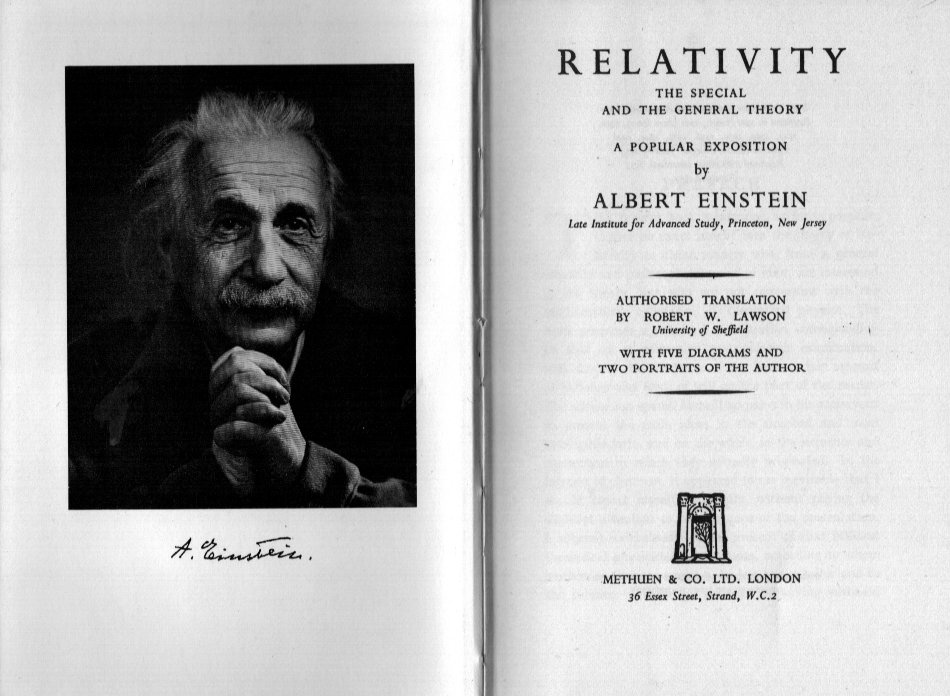Edited by studiot
spelling

##### Share on other sites

1 minute ago, Strange said:

You seem to have picked up an impressive number of misunderstandings in that time

Indeed, tell me. I read all kinds of alogical explanations by people that pretend to understand Special Relativity.

##### Share on other sites
50 minutes ago, vanholten said:

Einstein is saying a lot more than that the speed of light is the same in both directions.

Right, he is saying that the speed of light in both directions is the same with respect to any inertial frame as measured from that frame.

So in the following example we have two observers. One standing along the tracks and the other traveling along the tracks in a railway car.

Two flashes are emitted from two points along the tracks that are equal distance from the track observer. the light from these flashes arrive at the midpoint observer at the same moment as the railway observer is passing him.  Thus both observers detect the light from the flashes at the same time.  Like this:

For the midpoint observer ( or anyone at rest with respect to the tracks) these flashes were emitted simultaneously, as shown by the expanding circles: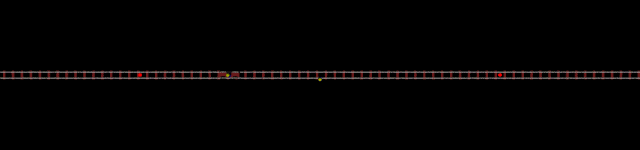However, for the railway car observer, events have to occur differently.  He still detects the light from both flashes simultaneously, and they arrive when he is adjacent to the track observer.

But unlike the track observer he has not remained halfway between the emission points the entire time.  He is not an equal distance from the emission points when either of the flashes was emitted.   But he must also measure the speed of light for each of the flashes as being the same relative to himself.    But since the distances each of these flashes travel relative to him are not the same, in order for the light of the flashes to reach him simultaneously, they must have left at different times. And the sequence of the events for him occur like this: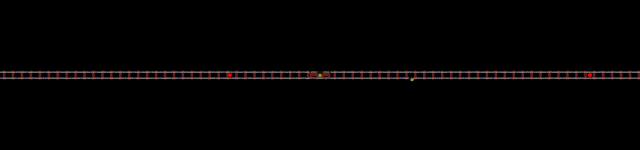For the track observer, the flashes are emitted simultaneously, but for the railway observer they are not.

This is the relativity of simultaneity: Events that are simultaneous in one inertial frame are not so according to another which in relative motion with respect to the first frame.

##### Share on other sites

Again nice simple but clear visualisations, janus. +1

Yes in the book I referred to Einstein also explains the implications of relativity of simultaneity for time and space.

Edited by studiot

##### Share on other sites

This is an attempt to demystify some issues concerning Special Relativity. The definition of simultaneity, part two.

The Rod AB.

"§ 2. On the Relativity of Lengths and Times:"-

“Taking into consideration the principle of the constancy of the velocity of light we find that

tB - tA = rAB / (c - v )                                t’A - tB = rAB / (c+v)

where rAB denotes the length of the moving rod—measured in the stationary system. Observers moving with the moving rod would thus find that the two clocks were not synchronous, while observers in the stationary system would declare the clocks to be synchronous.”

The diagram leaves no doubt that observers moving with the rod AB read out synchronous clocks, so why Einstein's remark concerning these formulas?

A calculation based on v = 0,5 c and the rod rAB having a length of 1 c . sec makes clear something very strange is happening.

tB - tA = (1c . sec) / 0,5 c     results in     tB - tA = 2 sec.

t’A - tB = (1c . sec) / 1,5 c   results in     t’A - tB = 0,666 sec.

Although working in opposite direction,  the formulas do not compensate for each other but have a very complex relation. Apparently something strange happened to the length of the rod.

“The length to be discovered by the operation (b) we will call “the length of the (moving) rod in the stationary system.” This we shall determine on the basis of our two principles, and we shall find that it differs from l.

Differs how? Length contraction or time dilation,  both or neither? I claim the formulas above are based on a biased perception that is actually a mistake.

The distance the light beam travels can be expressed as:

rAB + v . ( tB - tA )

Because all clocks on the moving rod and in the stationary system are synchronized, what Einstein assumed is:

tB - tA = { rAB + v . ( tB - tA )} / c

c . ( tB - tA ) = rAB + v . ( tB - tA )

c . ( tB - tA ) - v . ( tB - tA ) = rAB

( c - v ) . ( tB - tA ) = rAB

tB - tA = rAB / (c - v)

Mathematical it’s ok, however the following is allowed:

tB - tA = { rAB + v . ( tB - tA )} / c

rAB / c = { rAB + v . ( rAB / c )} / c

Rod AB is replaced by L

L / c =  { L +  v ( L /c )} /c

L  = L +  v ( L /c )

L . v / c = 0

L = 0  ,  c ≠ 0

v = 0  , c ≠ 0

L = rAB  ≠ 0

thus v = 0

This proves the formula only applies to the situation in which v = zero and therefore is pointless regarding the concept of relative motion.

Edited by vanholten

##### Share on other sites
On 15/11/2018 at 11:52 PM, vanholten said:

I read all kinds of alogical explanations by people that pretend to understand Special Relativity.

Yes there are some misguided popular explanations about.

It is also true that the modern development of the subject proceeds along a different path from the one Einstein originally took.

Further, textbooks and scientific papers are often quite brief in their explanations.

Einstein is particularly terse as shown by his introduction of "double index convention" abbreviation for the summation process.

So his statement you picked out

On 15/11/2018 at 8:11 PM, vanholten said:

Einstein

We assume that this definition of synchronism is free from contradictions, and possible for any number of points; and that the following relations are universally valid:

Is the result of quite a chain of reasoning, but it is all he says about it.

So here is a start to the process of unpicking the original 1905 paper and the chains of reasoning behind it.
These do not presuppose modern knowledge (as modern treatments often do) but leads us from simpler ideas to the theory.

So consider let us consider a journey from a point A to a point B in a given system of intertia - note there is only one system of inertia at this stage.
The velocity of travel from A to B is defined as the ratio of the distance AB and and the time of travel of the light.

Now working entirely within the one system of inertia we have no difficulty measuring the distance AB by laying out a standard measuring stick (for ease and without loss of generality, this comes to an exact whole number of these).
But measuring the difference in time is much more difficult. Indeed both the concepts of time itself and therefore velocity (which depends on time) become bogged down in circular arguments and therfore meaningless unless the issue of simultaneity can be addressed as we shall see.

If t1 is the time of emission at A as read on a clock at A and t2 is read on arrival on another clock at B then (t2-t1) is only the time which light has taken to travel from A to B if there is a known correspondence between the two clocks at A and B. We call setting this correspondence to zero synchronising the clocks.

So, the all important question,  how do we do this?

Well one method is to employ a third clock which we place next to the clock at A (this is what is meant by Einstein's comment " It is possible for the observer at B to determine the times of events in the neighborhood of B" - (no special geometric arrangements of clocks are needed or mentioned) in his paper. We set the third clock to the same reading as A, whilst it is 'at' A and then transport it infinitesimally slowly to B. Once at B we set the clock at B to the same reading as this third clock. We can then move this clock to other places and repeat, thereby ensuring that we have as many clocks as we wish distributed where we wish to make measurements simultaneous with our clock at A, all in the one single inertial system.
A variation (simplification) occurs if we only have points A and B since we can simply origianlly place the B clock at A, sychronise it, and then move it as described to B.

Einstein assumed that this theoretical process of movement would work satisfactorilly, (that is would not change the synchronisation of the travelling clock) but he did not prove it.
Proof was left to Eddington and you will find his prooof in his book "The Mathematical Theory of Relativity" on page 28.
This proof relies on the calculus of small quantities, but of course the actual taking and interpretation of such measurements were his field.

A more modern method would be to use a very fast 'time signal' sent out from A to B eg a light signal.
If the signal was sent from A when time  = t1 = zero, we would then set the clock at B to t2 = AB/v where v is the velocity of the time signal.

But then we would have to know the velocity and propagation characteristics of that time signal.

But to know the velocity of the time signal or the effect of transport on a calibrating clock we would need the know the other.

That is to know the synchronous time we would need the velocity.
But to know the velocity we would need the synchronous time.

Both the above methods end in a circular argument, and indeed all methods of the regulation of multiple clocks end in such a difficulty.

This is why (all too briefly), Einstein states that we must define the concept of simultaneity.

If continuing this is of interest, next time we can look at the way out of this impasse, by means of using a single clock and a closed polygon path.

##### Share on other sites
2 hours ago, studiot said:

Einstein is particularly terse as shown by his introduction of "double index convention" abbreviation for the summation process.

I have never found his explanations particularly clear. I’m not sure why so many people seem to start with his writings on the subject.

##### Share on other sites
47 minutes ago, Strange said:

I have never found his explanations particularly clear. I’m not sure why so many people seem to start with his writings on the subject.

Yes, he generally wrote for his peers (those who are at least as clever as he was).

A small very select band.##### Share on other sites

Let me emphasize that no chain of reasons ending in Einstein definition of simultaneity can retrospectively justify the inevitable v = zero outcome of the equations,  from which all other calculations in § 3 and further are derived.         tB - tA = rAB /(c - v )             and                       t’A - tB = rAB / (c + v)     and       thus      v = 0

The for special relativity devastating prove of the twin equations resulting in zero velocity, also handover the clue on how to interpreted the second postulate. Evaluating simultaneity doesn't make this go away, but if you like I will give it a try.

On 28-11-2018 at 3:31 PM, studiot said:

Well one method is to employ a third clock which we place next to the clock at A (this is what is meant by Einstein's comment " It is possible for the observer at B to determine the times of events in the neighborhood of B" - (no special geometric arrangements of clocks are needed or mentioned) in his paper. We set the third clock to the same reading as A, whilst it is 'at' A and then transport it infinitesimally slowly to B. Once at B we set the clock at B to the same reading as this third clock. We can then move this clock to other places and repeat, thereby ensuring that we have as many clocks as we wish distributed where we wish to make measurements simultaneous with our clock at A, all in the one single inertial system.
A variation (simplification) occurs if we only have points A and B since we can simply origianlly place the B clock at A, sychronise it, and then move it as described to B.

Einstein assumed that this theoretical process of movement would work satisfactorilly, (that is would not change the synchronisation of the travelling clock) but he did not prove it.
Proof was left to Eddington and you will find his prooof in his book "The Mathematical Theory of Relativity" on page 28.
This proof relies on the calculus of small quantities, but of course the actual taking and interpretation of such measurements were his field.

Do you really think the solution to this problem lies in infinitesimally slow transport of clocksYou realize that infinitesimally slow transport results in infinitely long transport time. Meanwhile let me continue with a couple of straight forward calculations that obey Einstein's paper definition of simultaneity and do allow velocity to be a meaningful factor.

From "§ 1. Definition of Simultaneity"

“In agreement with experience we further assume the quantity

2AB / (t’A - tA) = c

to be a universal constant—the velocity of light in empty space.”

All distances concerning the motion of the rod AB are measured within the time interval t’A - t A. The equation shows that the length of rod AB is accounted for twice in relation to the universal constant - the velocity of c in empty space. And in accordance with Einsteins definition the two clocks synchronize if:

t B − t A = t A − t B

The completed time interval concerning rAB in motion is

tB - tA  +  t’A - tB

During the first timespan tB - tA , rod AB travels the distance

Dr  = v . ( tB - tA )

To reach B the photon emitted at A needs to travel the distance Dp.

At this point we meet the starting calculations of the twin equations in the previous post:

rAB + v . ( tB - tA )

The distance the photon travels within a stationary rod AB is  c . ( tB - tA )

The forward distance traveled by the photon is

Dpf =  c . ( tB - tA ) + v . ( tB - tA )

Dpf= ( c + v ) ( tB - tA )

on the way back the distance is

Dpb =  (c - v)  (t’A - tB)

The total distance traveled by the photon is

D = ( c + v ) ( tB - tA ) + (c - v) (t’A - tB)

( c + v ) (AB/c ) + (c - v) ( AB/c)

2AB

and matches

“In agreement with experience we further assume the quantity

2AB / (t’A - tA) = c

to be a universal constant—the velocity of light in empty space.”

Which for all observers has to be true according to the second postulate. These calculations do not violate “t B − t A = t ′A − t B” and support what Einstein wrote:-  "while observers in the stationary system would declare the clocks to be synchronous.” So far so good. Now let’s take a look at the observers moving with the moving rod AB. In their perception the velocity of the rod AB is v = 0.  The distance traveled by the photon forward equals the distance backwards.

D = ( c + 0 ) ( tB - tA ) + (c - 0) (t’A - tB)

matching

2AB / (t’A - tA) = c

Which for all observers has to be true according to the second postulate. Apparently the calculations apply to either the stationary system and to the system in motion, and match the definitions of simultaneity. So it remains unclear why Einstein wrote:  Observers moving with the moving rod would thus find that the two clocks were not synchronous”.

Next: "The second postulate:

2. Any ray of light moves in the “stationary” system of co-ordinates with the determined velocity c, whether the ray be emitted by a stationary or by a moving body."

This postulate is difficult to grasp because it says that any observer in motion or stationary compared to a light source measures the light to travel at c relative to himself. Against logic it implies that two observers in relative motion, each measure the light beams from one and the same light source to travel at c relative to themselves.

But what if we interpreter the postulate as follows, then what happens? In a system of coordinates any ray of light is measured to be emitted at the definite velocity c regardless of the relative motion of the light source it originates from.

It makes perfect sense and remains a valid rejection of the aether theory. But in this interpretation it can't be taken for granted that the velocity of light is measured to be a universal constant, so that must be fixed.

To do so we should take another look at rod AB and the calculus of small quantities you mentioned. The velocity of the rod rAB relates to the timespan t’A - tA . This implies that the own length of rAB limits the extent the rod travels during that same time span. Because the motion of rAB is one directional and v < c the distance D within t’A - tA never exceeds 2x the length of rod rAB per definition.

"2AB / (t’A - tA) = c"

and D= 2ABv/c

If AB is chosen to be infinitesimally short, D also approaches zero. Within the accompanying time span the light source is ~ stationary to all observers regardless of their own relative motion. It looks like Einstein indeed chose rAB represented by x' to be infinitesimally small.

§ 3: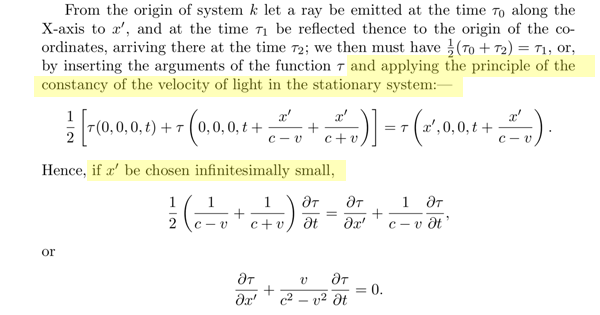Perhaps a more to the point approximation of the narrowest window of opportunity in which velocity can manifest is the emission event of a photon. That emission event is compliant with the average wavelength of light ~ 10-7 m. ( c= λ . f )

“In agreement with experience we further assume the quantity

2AB / (t’A - tA) = c

to be a universal constant—the velocity of light in empty space.”

It appears to me that the quantity  2AB / (t’A - tA) = c  inevitably has to refer to the reasonable assumption that lightwaves are quantities of simultaneity and thus to quantum mechanics and the Planck Constant.

At an astronomical scale it is fair to say c + v approximates c - v thus v approximates 0 or even v = 0. The outcome v = 0 brings us back to the twin equations mentioned in the header of this post. From those equations Einstein derived length contraction and time dilation.

Length contraction    L = L0 √ (1-(v/c)²)   or         L = L 0/ γ

Time dilation         Δ t’ = Δ t/ √ (1-(v/c)²)  or     Δ t’ = Δ t . γ

L . Δ t’ = L 0 . Δ t

(L 0/ γ)  .  Δ t . γ =  L 0 . Δ t

(1/ γ ) . γ =  1

Length contraction shortens the rod and according to D= 2ABv/c as such it travels a shorter distance. Meanwhile due to time dilation it takes more time to bridge that shorter distance. Expressed in m/s the velocity entered in the equations remains unchanged and since the velocity of light is a constant, the relative distance and the length of the rod measured by clocks remain unchanged. At their best TD and LC refer to frequency and wavelength in relation to constant velocity, but nevertheless are doomed to cancel each other out when attempting to produce spacetime curvature.

Now let's take a look at both postulates.

"1. The laws by which the states of physical systems undergo change are not affected, whether these changes of state be referred to the one or the other of two systems of co-ordinates in uniform translatory motion.

2. Any ray of light moves in the “stationary” system of co-ordinates with the determined velocity c, whether the ray be emitted by a stationary or by a moving body."

My summary: Any light emitted propagates a the definite velocity c away from the light source regardless of the relative motion of the light-source. For the proper measurement of the velocity of a photon the light source compares to be stationary to any inertial frame of reference. In my opinion the second postulate is no complement but should rather be an exception to the first postulate. In short: Galilean Relativity does not apply to the measurement of the propagation velocity of light. It is about the uncertain relation between measurement, location and impulse. I gather this intriguing issue is finally solved.

##### Share on other sites
50 minutes ago, vanholten said:

Let me emphasize that no chain of reasons ending in Einstein definition of simultaneity can retrospectively justify the inevitable v = zero outcome of the equations,  from which all other calculations in § 3 and further are derived.         tB - tA = rAB /(c - v )             and                       t’A - tB = rAB / (c + v)     and       thus      v = 0

The for special relativity devastating prove of the twin equations resulting in zero velocity, also handover the clue on how to interpreted the second postulate. Evaluating simultaneity doesn't make this go away, but if you like I will give it a try.

This was rather confusing.

I am also a bit confused between your thread and conjuror's thread about a similar matter and may have mixed them up a bit.

Anyway you haven't answered my question and my development was meant eventually to show why the postulates of SR are necessary.
It did not start with them, and in fact it specifically excluded them.

So it would eventually answer your questions, but since you don't seem to want to pursue it never mind.

## Create an account

Register a new account